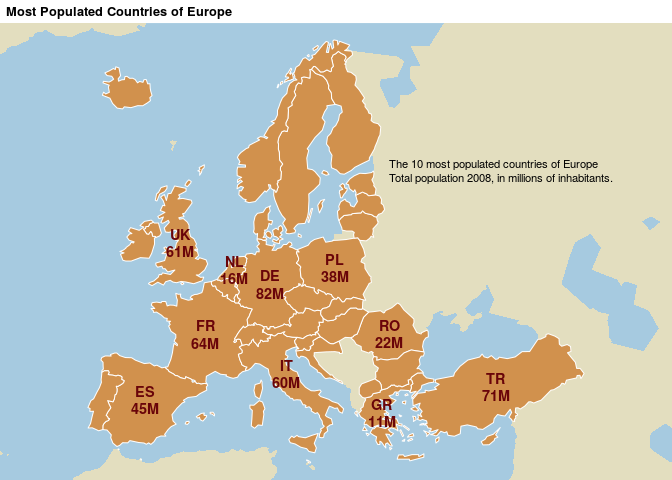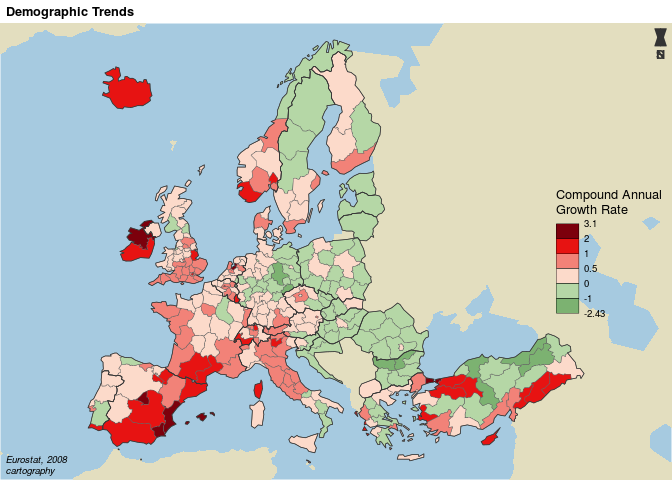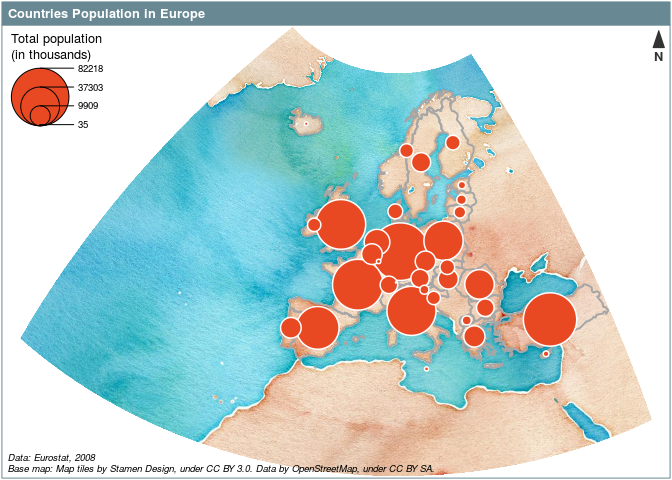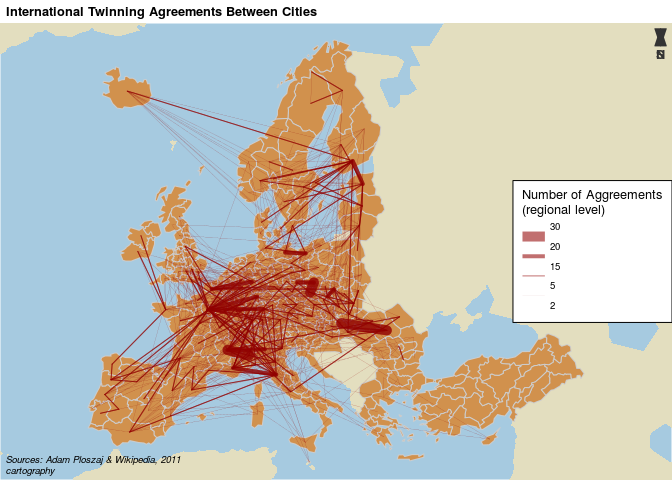# Label Map

``````library(cartography)
data(nuts2006)
# set margins
opar <- par(mar = c(0,0,1.2,0))

# Layout plot
layoutLayer(title = "Most Populated Countries of Europe", # title of the map
author = "",  # no author text
sources = "", # no source text
scale = NULL, # no scale
col = NA, # no color for the title box
coltitle = "black", # color of the title
frame = FALSE,  # no frame around the map
bg = "#A6CAE0", # background of the map
extent = nuts0.spdf) # set the extent of the map

# Non European space
plot(world.spdf, col  = "#E3DEBF", border=NA, add=TRUE)
# European (EU28) countries
plot(nuts0.spdf, col = "#D1914D",border = "white", lwd=1, add=TRUE)

# Selection of the 10 most populated countries of Europe
dflab <- nuts0.df[order(nuts0.df\$pop2008, decreasing = TRUE),][1:10,]
# Label creation
dflab\$lab <- paste(dflab\$id, "\n", round(dflab\$pop2008/1000000,0), "M", sep ="")

# Label plot of the 10 most populated countries
labelLayer(spdf = nuts0.spdf, # SpatialPolygonsDataFrame used to plot he labels
df = dflab, # data frame containing the lables
txt = "lab", # label field in df
col = "#690409", # color of the labels
cex = 0.9, # size of the labels
font = 2) # label font

text(x = 5477360, y = 4177311, labels = "The 10 most populated countries of Europe
Total population 2008, in millions of inhabitants.", cex = 0.7, adj = 0)````````````
par(opar)``````

# Choropleth Map

``````library(cartography)
data(nuts2006)
# set margins
opar <- par(mar = c(0,0,1.2,0))

# Compute the compound annual growth rate
nuts2.df\$cagr <- (((nuts2.df\$pop2008 / nuts2.df\$pop1999)^(1/9)) - 1) * 100

# Set a custom color palette
cols <- carto.pal(pal1 = "green.pal", # first color gradient
n1 = 2, # number of colors in the first gradiant
pal2 = "red.pal", # second color gradient
n2 = 4) # number of colors in the second gradiant

# Plot a layer with the extent of the EU28 countries with only a background color
plot(nuts0.spdf, border = NA, col = NA, bg = "#A6CAE0")
# Plot non european space
plot(world.spdf, col  = "#E3DEBF", border=NA, add=TRUE)

# Plot the compound annual growth rate
choroLayer(spdf = nuts2.spdf, # SpatialPolygonsDataFrame of the regions
df = nuts2.df, # data frame with compound annual growth rate
var = "cagr", # compound annual growth rate field in df
breaks = c(-2.43,-1,0,0.5,1,2,3.1), # list of breaks
col = cols, # colors
border = "grey40", # color of the polygons borders
lwd = 0.5, # width of the borders
legend.pos = "right", # position of the legend
legend.title.txt = "Compound Annual\nGrowth Rate", # title of the legend
legend.values.rnd = 2, # number of decimal in the legend values

# Plot a layer of countries borders

# Layout plot
layoutLayer(title = "Demographic Trends", author = "cartography",
sources = "Eurostat, 2008", frame = TRUE, col = NA,
scale = NULL,coltitle = "black",
south = TRUE) # add a south arrow````````par(opar)``

# Base Map and Proportional Symbols

``````library(cartography)
data(nuts2006)
# set margins
opar <- par(mar = c(0.1,0.1,1.3,0.1))

## Plot Stamen tiles (using OpenStreetMap data) as basemap
EUosm <- getTiles(spdf = nuts0.spdf, type = "stamenwatercolor", zoom = 4)
# Plot the tiles
tilesLayer(EUosm)

# Plot countries
plot(nuts0.spdf, border = "grey65", lwd = 2, add=TRUE)

# Plot countries population
nuts0.df\$pop <- nuts0.df\$pop2008 / 1000
propSymbolsLayer(spdf = nuts0.spdf, # SpatialPolygonsDataFrame of the countries
df = nuts0.df,  # data frame of the regions
var = "pop",  # population
symbols = "circle", # type of symbol
border = "white", # color of the symbols borders
lwd = 1.5, # width of the symbols borders
legend.pos = "topleft",
legend.title.txt = "Total population\n(in thousands)")
# Layout plot
layoutLayer(title = "Countries Population in Europe",
sources = "Data: Eurostat, 2008",
author = "Base map: Map tiles by Stamen Design, under CC BY 3.0. Data by OpenStreetMap, under CC BY SA.",
scale = NULL, frame = TRUE,
col = "#688994") # color of the frame````````par(opar)``

``````library(cartography)
data(nuts2006)
# set margins
opar <- par(mar = c(0,0,1.2,0))

# Create a link layer from the twincities data frame
##      i    j fij
## 1 DE14 AT11   1
## 2 DE21 AT11   1
## 3 DE23 AT11   1
## 4 DE26 AT11   2
## 5 DE91 AT11   1
## 6 DEB3 AT11   1
# twincities contains links between Nuts 2 regions
# ?twincities.df
twincities.spdf <- getLinkLayer(spdf = nuts2.spdf, # SpatialPolygonsDataFrame of Nuts2
df = twincities.df) # links data frame
class(twincities.spdf)
##  "SpatialLinesDataFrame"
## attr(,"package")
##  "sp"

# Plot a layer with the extent of the EU28 countries with only a background color
plot(nuts0.spdf, border = NA, col = NA, bg = "#A6CAE0")
# Plot non european space
plot(world.spdf, col  = "#E3DEBF", border=NA, add=TRUE)
# Plot Nuts2 regions
plot(nuts2.spdf, col = "#D1914D",border = "grey80", add=TRUE)

df = twincities.df, # data frame of the links
spdfids = "i", # identifier of starting points in spdf
spdfide = "j", # identifier of ending points in spdf
dfids = "i", # identifier of starting points in df
dfide = "j", # identifier of starting points in df
var = "fij", # name of the variable used to plot the links widths
breaks = c(2,5,15,20,30), # list of breaks
lwd = c(0.1,1,4,10), # list of widths
col = "#92000090", # color of the links
legend.pos = "right", legend.frame = TRUE,
legend.title.txt = "Number of Aggreements\n(regional level)",

# Plot the layout
layoutLayer(title = "International Twinning Agreements Between Cities",
author = "cartography", sources = "Sources: Adam Ploszaj & Wikipedia, 2011",
scale = NULL, south = TRUE, frame = TRUE, col = NA,
coltitle = "black")````````par(opar)``

# Proportional Symbols and Choropleth Map

``````library(cartography)
data(nuts2006)
# set margins
opar <- par(mar = c(0,0,1.2,0))

# Compute the compound annual growth rate
nuts2.df\$cagr <- (((nuts2.df\$pop2008 / nuts2.df\$pop1999)^(1/9)) - 1) * 100

# Plot a layer with the extent of the EU28 countries with only a background color
plot(nuts0.spdf, border = NA, col = NA, bg = "#A6CAE0")
# Plot non european space
plot(world.spdf, col  = "#E3DEBF", border=NA, add=TRUE)
# Plot Nuts2 regions
plot(nuts2.spdf, col = "grey60",border = "white", lwd=0.4, add=TRUE)

# Set a custom color palette
cols <- carto.pal(pal1 = "blue.pal", n1 = 2, pal2 = "red.pal", n2 = 4)

# Plot symbols with choropleth coloration
propSymbolsChoroLayer(spdf = nuts2.spdf,
df = nuts2.df,
var = "pop2008", #  field in df to plot the symbols sizes
inches = 0.1, # set the symbols sizes
var2 = "cagr", #  field in df to plot the colors
col = cols, # symbols colors
breaks = c(-2.43,-1,0,0.5,1,2,3.1), # breaks
border = "grey50",  # border colors of the symbols
lwd = 0.75, # symbols width
legend.var.pos = "topright", # size legend position
legend.var.values.rnd = -3, # size legend value roundinf
legend.var.title.txt = "Total Population", # size legend title
legend.var.style = "e", # size legend type
legend.var2.pos = "right", # color legend position
legend.var2.title.txt = "Compound Annual\nGrowth Rate") # legend title

# layout
layoutLayer(title = "Demographic trends, 1999-2008", coltitle = "black",
sources = "Eurostat, 2011", scale = NULL,
author = "cartography", frame ="", col = NA)``````